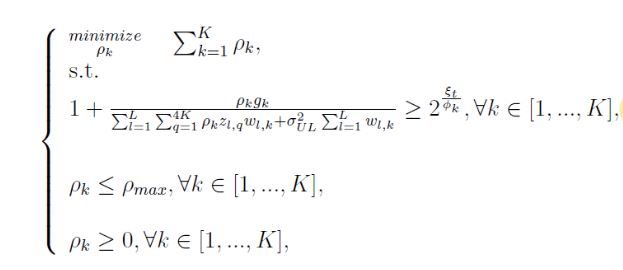# Prove the optimal solution of linear program

this equation is a LP. I have a some question.How can I briefly prove with mathematical relations that this LP relation gives the optimal solution?

I’m not sure exactly what the question is, but it is not specific to CVX, nor is this a homework site.

Consider posting a question, demonstrating some solution effort by you, at https://or.stackexchange.com/ or https://math.stackexchange.com/ .